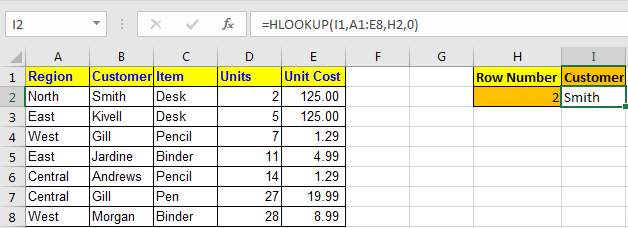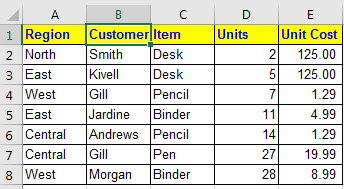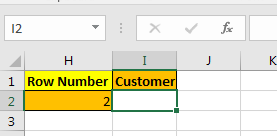# How to Use HLOOKUP Function in Excel

HLookup Stands for Horizontal Lookup. Same as Vlookup, it looks for a value in a table but Row wise, not column wise.Syntax of HLOOKUP

=HLOOKUP(lookup value, table array, row index number, [range_lookup] )
• lookup value : The value you are looking for.
• Table Array : The table in which you are looking for the value.
• Row Index Number : The row number in Table from which you want retrieve data.
• [range_lookup] : its the match type. 0 for exact match and 1 for approximate match.

Let’s understand it by an example:

## Excel HLOOKUP Example:

I have this data:And I have this queryI want to retrieve data from table in I2 by just changing heading in I1 and row number in H2.

To do this, I write this HLOOKUP formula in cell I2

=HLOOKUP(I1,A1:E8,H2,0)

Now whenever you change heading, the HLOOKUP formula will show data of that heading.The above HLOOKUP function will look for exact match in the first row of table and will return the value from given row number.

If you want an approximate match use 1 instead of 0 at range_lookup.

Pro Notes:

• Always sort first row your data in ascending order while looking for approximate match.

HLOOKUP is very useful if you are searching for data row-wise. It can be combined with INDEX function to get data using headings row titles.

HLOOKUP function is available in Excel 2016, 2013, 2010 and 2007 and maybe in older versions too (I never used them).

Related Articles:

How to use the Excel VLOOKUP Function